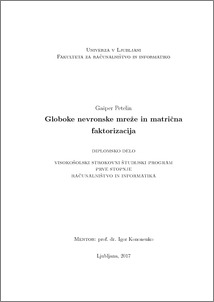# Deep neural networks and matrix factorization

Gašper Petelin (2017) Deep neural networks and matrix factorization. EngD thesis.Preview
PDF

## Abstract

This thesis proposes a new data-driven method for neural network weight initialization, where input data matrix is first factorized into multiple smaller matrices, each containing a summarized version of original data. Multiple shallow neural networks are then trained using acquired smaller matrices to learn simple functions, mapping one summarized data matrix into another, usually smaller matrix. One last shallow neural network is added to map the last summarized data matrix into their respective class labels if we are trying to classify data into multiple classes. On the other hand, if we are dealing with regression problem, the last neural network represents a simple mapping from summarized data into a single real value. All shallow neural networks are then combined into one deep network and additionally trained as a single neural network. The proposed method usually works better for deep neural networks, where random initialization often overfits or learns very slowly. To evaluate and compare the proposed method with other initialization methods, two datasets were used. The MNIST dataset was used to test classification accuracy and the Jester jokes dataset was used to predict ratings for individual jokes.

Item Type: Thesis (EngD thesis)
Keywords: deep neural networks, classification accuracy, matrix factorization, archetypal analysis, weight initialization
Number of Pages: 57
Language of Content: Slovenian
Mentor / Comentors:
Name and SurnameIDFunction
prof. dr. Igor Kononenko237Mentor